# What Are Data Structures? Trees

## What is a data structure?

A data structure is a method for organising a set of data. The structure is defined by how the data is stored and how operations, such as data access, insertion and deletion, are performed on the stored data. Data structures are essential tools for programmers, as each structure has a set of benefits that make it useful for solving certain types of problem.

## Abstract data structures

A tree is an abstract data structure. The definition of the structure is only a general idea and doesn't contain low level specifics such as how the data is stored in memory. This is in contrast to more fundamental data structures, such as arrays and linked lists, which have strict requirements for how their storage is implemented. Indeed, a tree can be implemented around an underlying array or through a linked list of nodes.

## General idea

A tree consists of a hierarchy of tree nodes, with each node storing a single piece of data. Each node can be connected to other nodes through tree edges. The only requirement is that the tree cannot contain any cycles.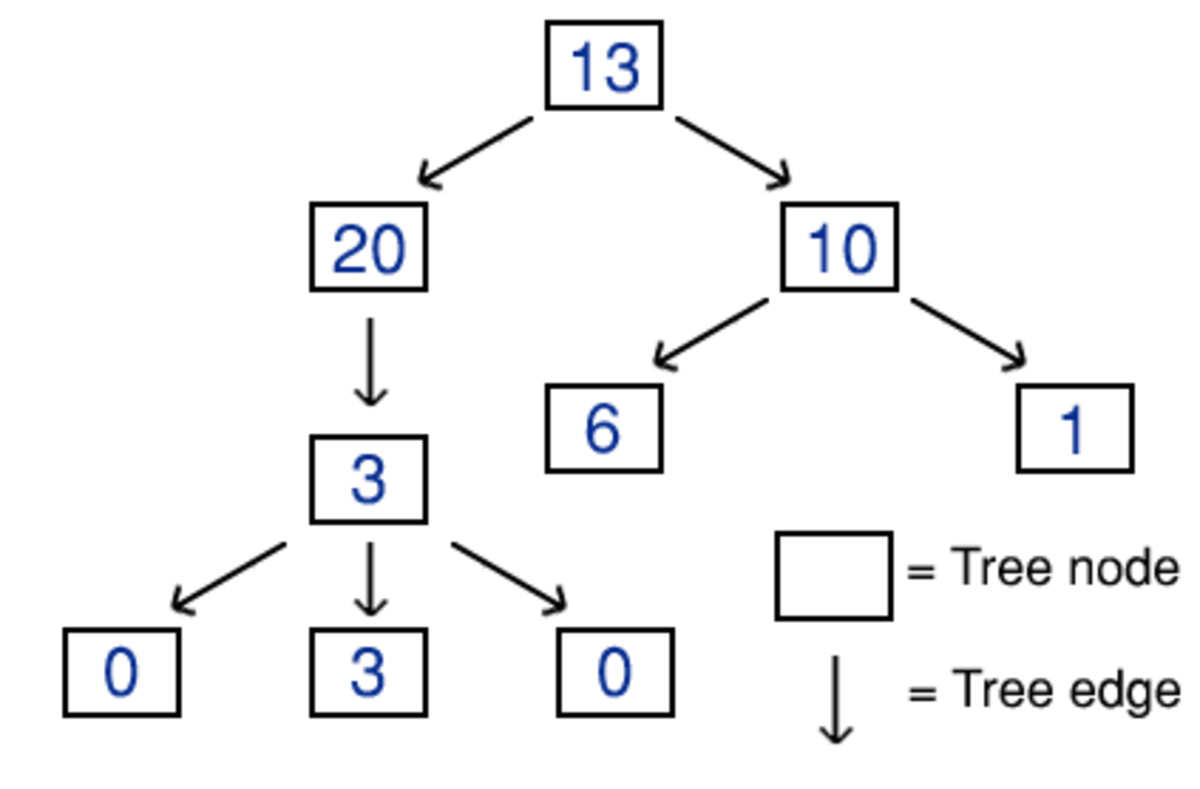An example of a tree that is storing a collection of integers. Stored data is represented by the colour blue.

There are two ways to look at a tree structure. A tree can be viewed as a whole, a single, ordered structure (as we do when looking at a tree diagram). However, this isn't how the tree is typically viewed when it is being implemented in code. The tree is usually viewed as a node that is possibly connected to multiple smaller trees. Each smaller tree consists of a node that is possibly connected to multiple even smaller trees and so on. This repetitive viewpoint naturally leads to recursive programming.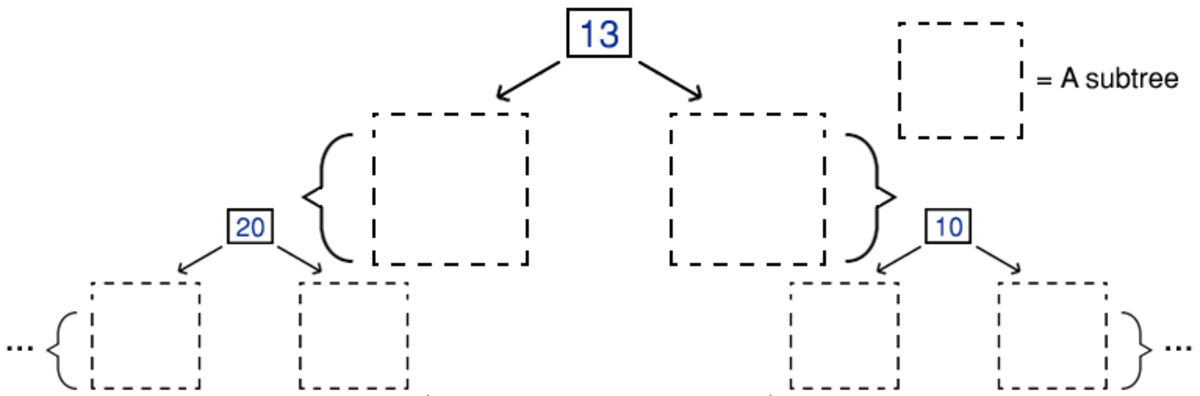An image demonstrating how a tree can be viewed as a series of individual nodes that have further smaller trees (subtrees) following on from them.

## Terminology

• Node - A location within the tree that stores a single piece of data. For example, this could be an array element or a linked list style node.
• Edge - A connection between two nodes. For example, this could be a formula to convert between array indices or it could be a pointer.
• Root - The top node in the tree. The whole tree can be accessed by starting from the root node.
• Parent - The node connected to the current node that is one step up from the current node, travelling towards the root. Every node in the tree, except the root, has a single parent.
• Child - A node connected to the current node that is one step down from the current node, travelling away from the root.
• Siblings - A group of nodes that all share the same parent.
• Leaf - A node that has no children. The bottom of the tree consists of leaf nodes.
• Ancestor - A node that can be reached from the current node by repeatedly moving from child to parent.
• Descendant - A node that can be reached from the current node by repeatedly moving from parent to child.
• Path - A specific sequence of nodes and edges that connects an ancestor to a descendant.
• Depth (of a node) - The number of edges separating the node from the root.
• Level (of a node) - The node's depth plus one.
• Level-order - A specific ordering of the values within a tree. Level-order starts on level one and moves downwards, moving from left to right within each level.
• Subtree - A node (other than the root node) and all of its descendants. Essentially, a smaller tree that is part of contained within the larger tree.
• Degree (of a node) - The number of subtrees that branch off the node. This is equal to the number of children (as each child can be thought of as a root to a possible subtree).
• Degree (of the tree) - The maximum degree of nodes within the tree.

## Binary tree

A binary tree is a tree that has a degree of two. Each parent is only allowed to have a maximum of two children, commonly referred to as the left and right child.

## Binary search tree (BST)

A binary search tree is a binary tree that is arranged in a specific order. The parent node contains data that is greater than all of the data in the left subtree and less than all of the data in the right subtree. Greater than and less than are general ordering concepts that can be defined for data types other than numbers.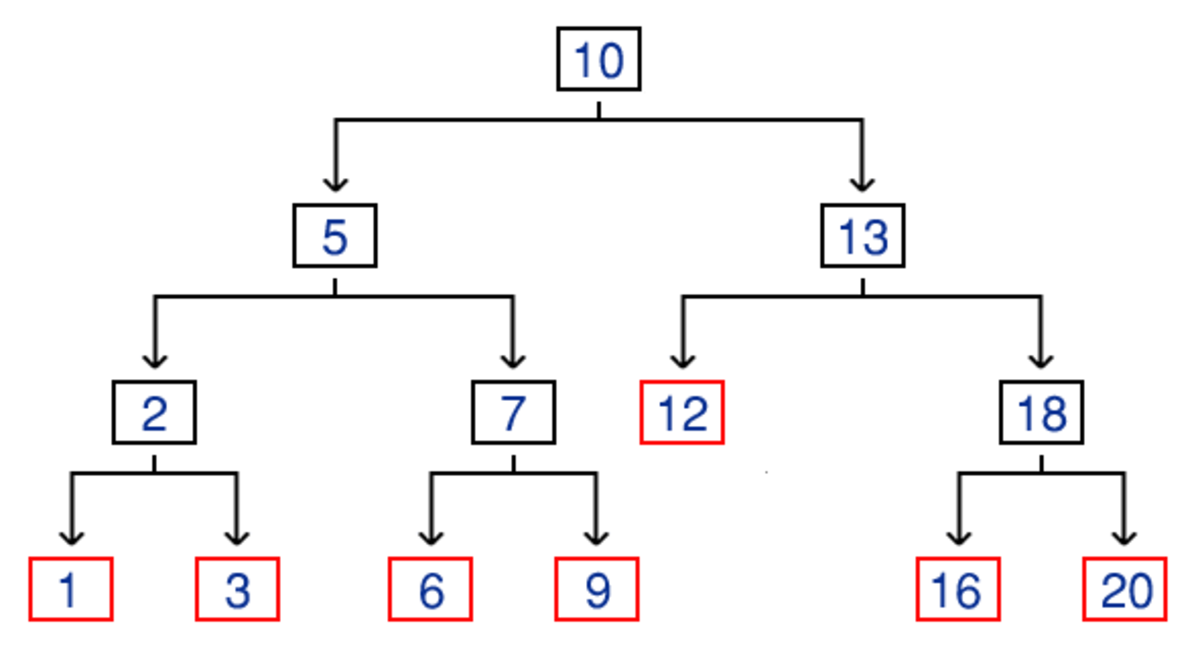An example of a binary search tree that is storing a collection of integers. Leaf nodes are coloured red to illustrate where the tree ends.

Having a specified order speeds up the process of searching for values within the tree. At each step of a search half of the tree can be ignored, analogous to a binary search. The average efficiency for standard operations (inserting, searching and deleting) is only O(log2(n)). However, this is dependant on having a well balanced tree. A balanced tree has roughly the same number of nodes within the left subtree and right subtree of all non-leaf nodes. The most unbalanced BST would consist of exclusively left nodes or exclusively right nodes, i.e. a linked list. This worst case would reduce the efficiency of operations to O(n).

The above code declares a template BST class in C++. Each node stores a piece of data and pointers to the left and right child nodes. A pointer is directly stored for the root node. The constructor sets the root to a null pointer, indicating an empty tree. The destructor calls another private function. The find, insert and delete operations also act in a similar way. The private functions recursively implement the operation and the public functions act as a wrapper for these recursive functions, passing the root node as a parameter to begin the recursive process.

The recursive function for the destructor deallocates the memory for a single node. It does this by calling itself on the nodes children and then deallocating the node. This safely deallocates the memory for all nodes in the tree.

The recursive function for insertion calls itself on either the left or right child node (depending on the value of the new data) until it is passed a null pointer, indicating an empty space available for insertion. The insertion is trivial: a new node is allocated, the data is set to the user input and the children are set to null pointers.

The recursive function for finding a node traverses the tree in an identical manner to the insertion function. When it reaches a null pointer or finds the requested data it returns a pointer to the current node. The wrapper function compares this value to a null pointer to workout whether the value was found.

Scroll to Continue

The recursive function for the deletion of a node is the most complicated. First, it recursively traverses the tree to find the requested node, similar to the previous functions but also keeping track of the previously visited node, i.e. the current node's parent. It then calls another function to remove the current node. There are three types of node removal:

1. Remove a leaf node. This is simple, there are no children to worry about. Delete the leaf node and make sure the parent's pointer is reset to a null pointer.
2. Remove a node with only one child. Change the parent's pointer to point to the current node's child. The current node can then be deleted.
3. Remove a node with two children. Replace the node's data with the minimum value from the right subtree. Then call the deletion function for this minimum value within the right subtree. The removal of the minimum node is guaranteed to be one of the previous cases (because it can't have a left child).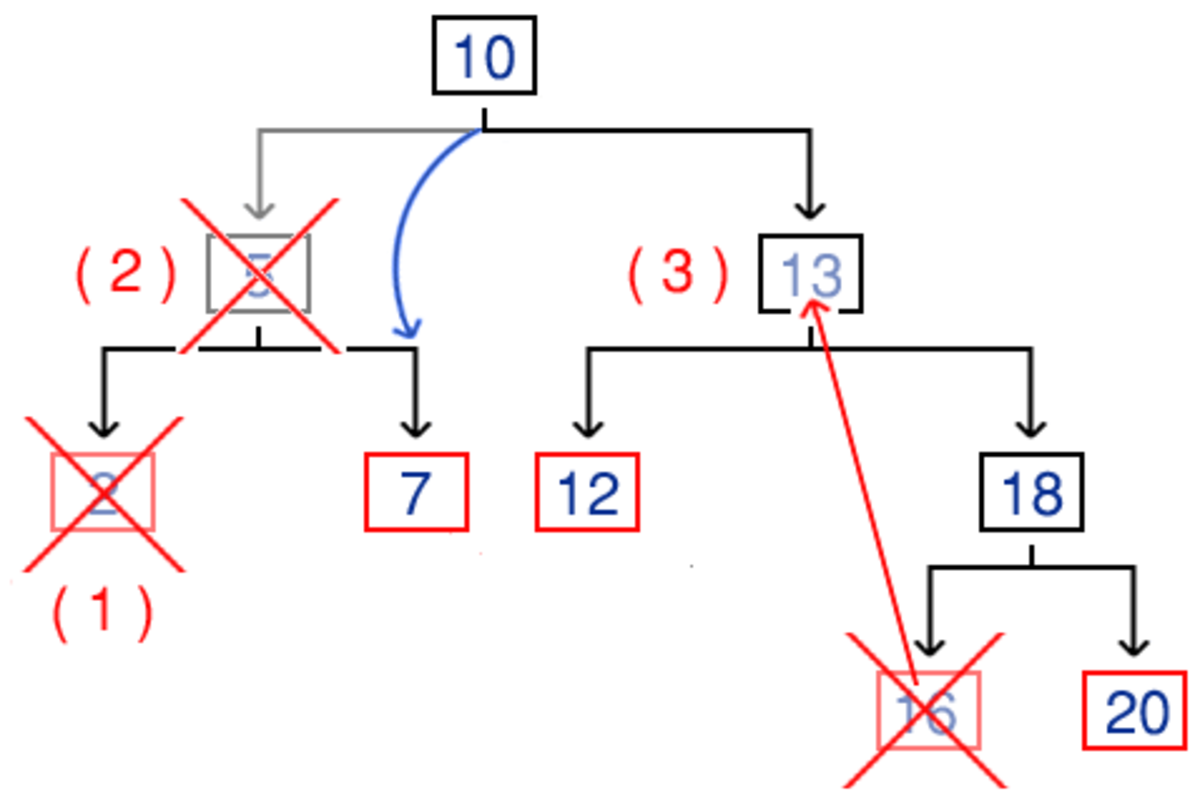A diagram showing the different types of BST deletion. The node next to the number in brackets is the node we call the deletion operation on. A red cross shows which node is actually deleted and a red arrow indicates the replacement of node data.

## Array implementation

Although less intuitive than the previous linked list based node structure, a tree could be stored by putting tree values within an array. The tree nodes could be stored in level order within the array. The edges that move from one node to another are then simple formulae, that calculate a new array index from the current index. The main problems with an array implementation are the need to deal with an array having a fixed size and empty gaps within the array for missing nodes, this is particularly bad for an unbalanced tree.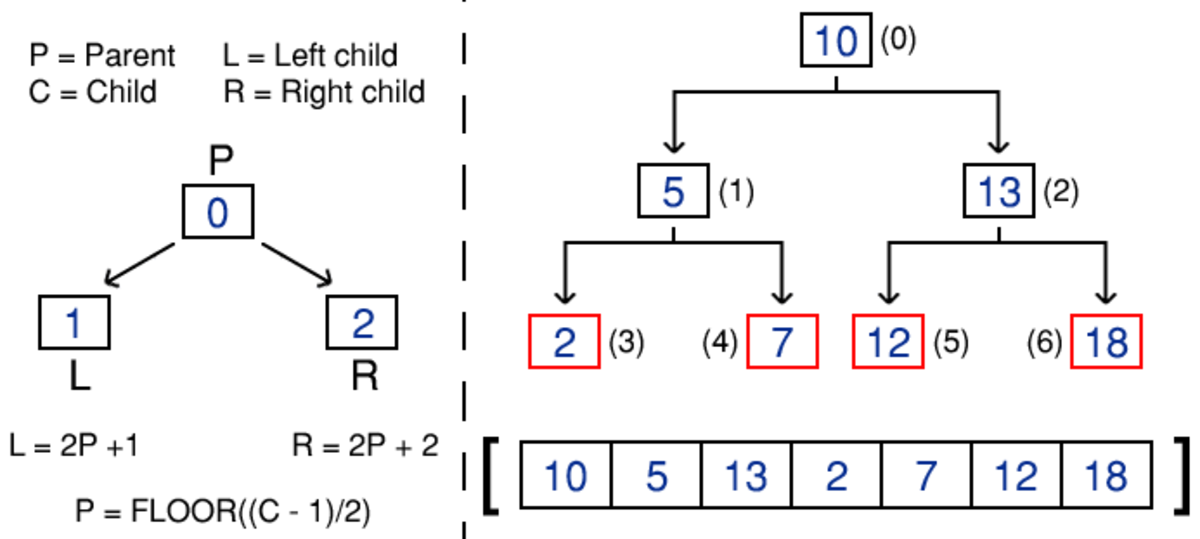On the left, the formulae for moving between the array indices of a parent node and its two children. On the right, an example of a BST stored in level-order within an array.

## Tries

A trie is a specific kind of tree that is used to store strings, commonly representing words. The general idea of a trie is that the different paths through a trie spell out different words stored by the trie. Each trie node stores a collection of data pairs; each pair has a character (that follows on from the current character) and a pointer to the next trie node. This could be stored as an array of pointers that has a length of 26, representing each possible alphabetical character. Another common implementation is to store the pairs in a hash table.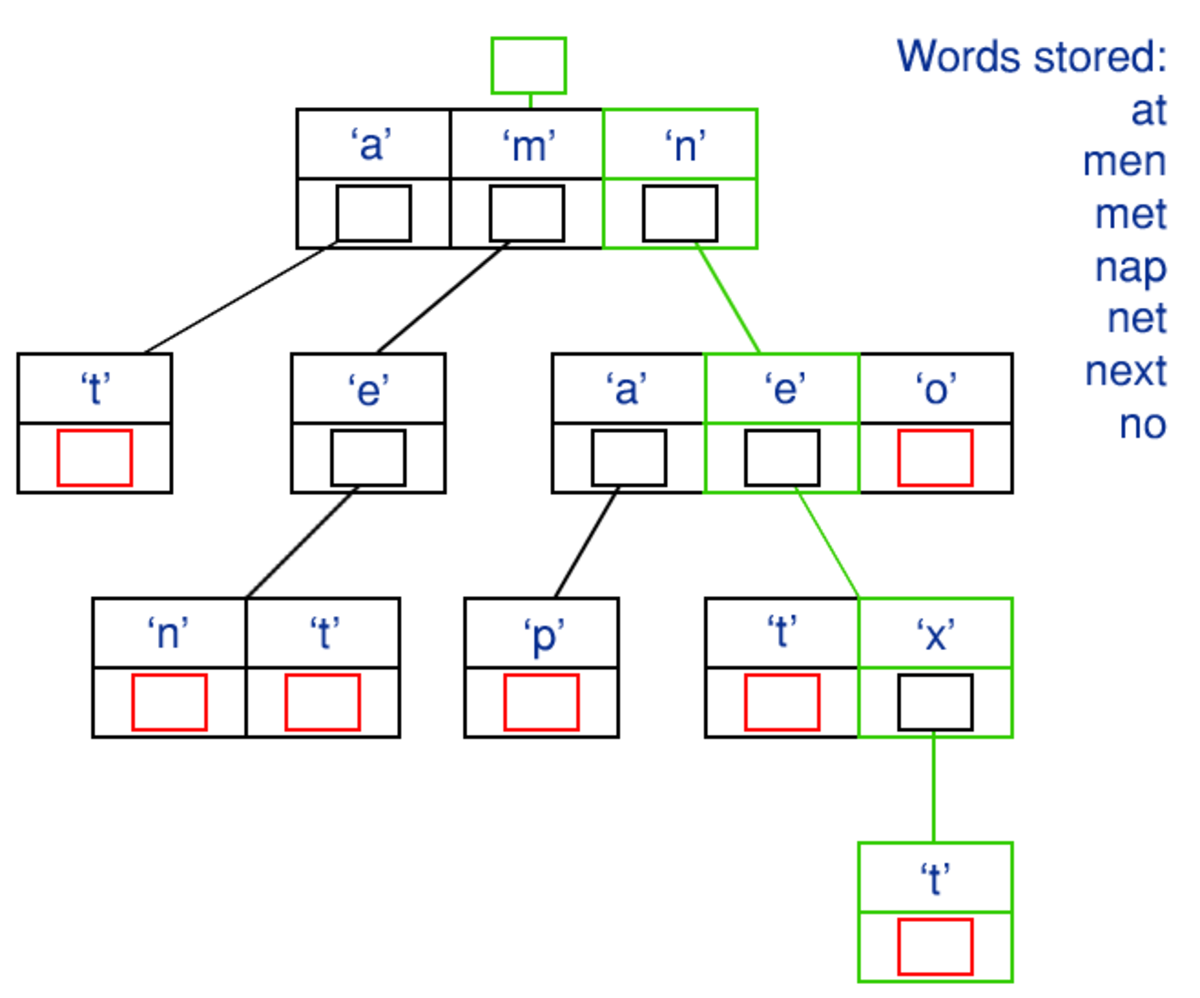An example trie that is used to store multiple short words. The nodes that signify the end of a word are highlighted red for clarity. A path that spells out the word 'next' when traversed is highlighted green.

The above code implements a trie class in C++. This implementation uses a hash table (the unordered_map from the STL) to store the possible next characters and the pointers to their associated tries. A count of the possible words that follow from the trie is also directly stored. The constructor creates an empty trie, with a count of zero and an empty hashtable. The destructor calls a private function that deletes all of a parent's child nodes, stored within the parent's hash table. This will recursively call itself to delete the whole tree of trie nodes (moving upwards from the leaves to the root).

There are four public member functions. There is a function to move onto one of the possible next characters. First, the hash table is searched for a user specified character. If this search is successful then the stored pointer to that character's trie is returned. If the character can't be found in the hash table, then a null pointer is returned.

The next function checks if a word is currently contained within the trie. It does this by iterating over each character in the user specified word. For each character, we attempt to move onto that character. If one of these moves fail, we return false. If we manage to successfully move onto every character then we have traversed the whole word and we return true.

The next function counts how many stored words begin with a partial string. It does this by traversing the trie along the characters of the user specified partial string, in an identical manner to the previous function. If the traversal fails at any character, we return a zero count. After a full traversal, the count stored by the final trie node is returned.

The final function is the most important, inserting a new word into the trie. First, we check that the word hasn't already been inserted as this means we don't have to do anything. Then we loop through each character in the word. The count is incremented for the current trie node and then we attempt to move onto the character. If this is unsuccessful, i.e. this character hasn't been stored yet, we need to add a new character pair to the previous trie node's hash table. A new trie node is dynamically allocated and its pointer is added to the hash table with the corresponding character.

## Uses

Like all other data structures, trees should be used when their attributes are suitable for the problem. Some examples being:

• The folders used by a computer's operating system will be stored in a tree. A folder structure is clearly hierarchical and well suited to being represented by a tree.
• Trees can be used to implement artificial intelligence. Possible scenarios would lead to a path of traversal and the final nodes would store decisions of what action the AI should take.
• Tries are very useful for common word based operations such as autocorrect, spell checking and input validation.
• A real life example of a tree is a family tree.

## Quiz

For each question, choose the best answer. The answer key is below.

1. Which type of tree node doesn't have a parent node?
• Root
• Leaf
2. In a BST, there is a parent node that stores the number 4, which child node would the number 6 be stored in?
• Left
• Right
3. What is the average efficiency of deletion from a BST?
• O(1)
• O(log(n))
• O(n)